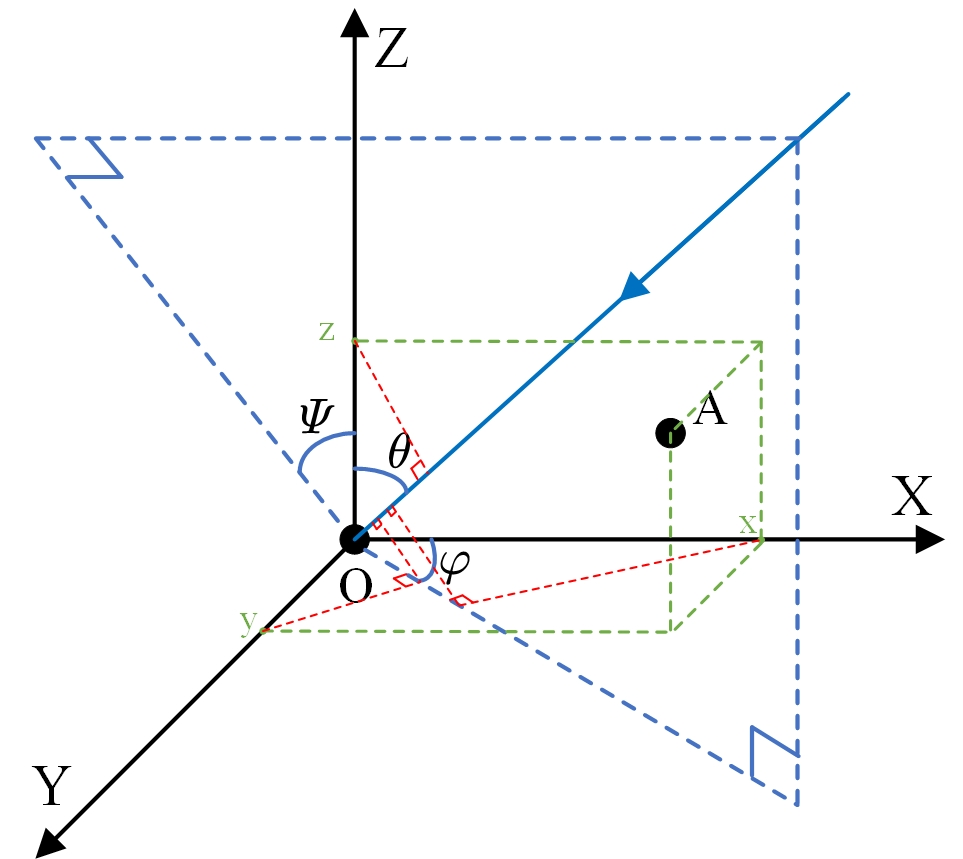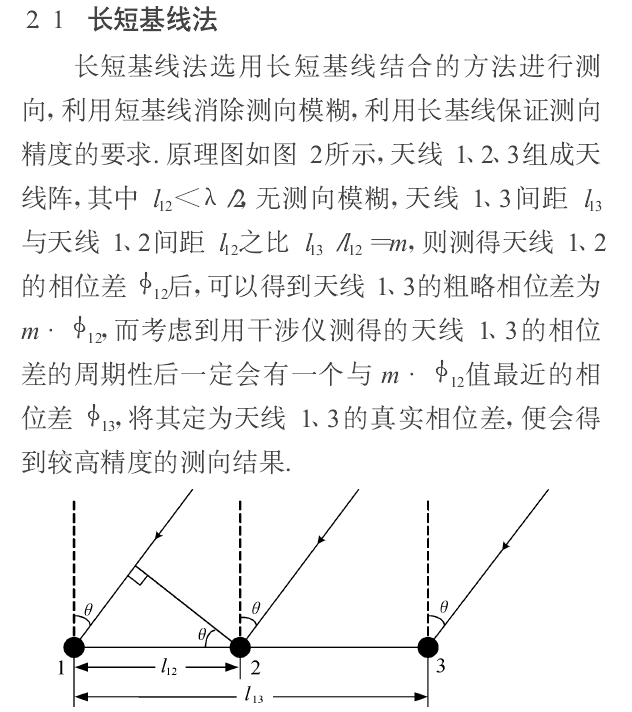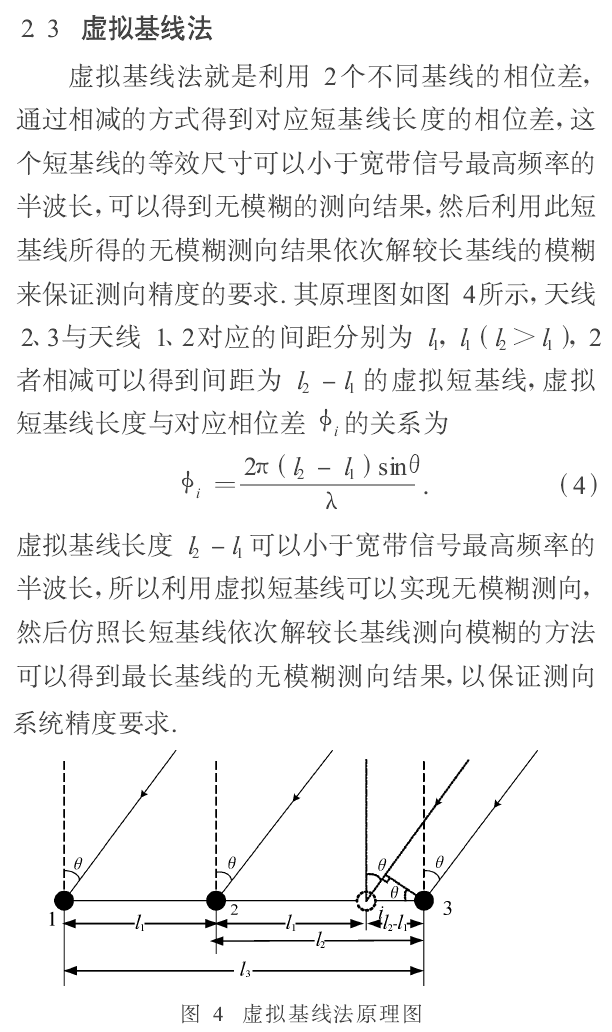# 对于干涉仪二维测向的一些要点

## 二维DOA估计要点tan ⁡ Ψ = tan ⁡ φ tan ⁡ θ \tan \varPsi =\frac{\tan \varphi}{\tan \theta}

Δ d = x cos ⁡ φ sin ⁡ θ + y sin ⁡ φ sin ⁡ θ + z cos ⁡ θ \Delta d=x\cos \varphi \sin \theta +y\sin \varphi \sin \theta +z\cos \theta

## 干涉仪估计要点

Δ ϕ = 2 π λ Δ d \Delta \phi =\frac{2\pi}{\lambda}\Delta d

#### 长短基线法：用于提高阵列测向精度#### 虚拟基线法：用于增大测向频率范围## 干涉仪用于二维DOA估计的要点

• 十字阵列一般以最中心的天线为原点，其他天线都布置在轴上。计算各阵元之间相位差的时候注意最好用正半轴天线的接收数据来与负半轴的天线做相关运算，保证计算出的相位差都是正数，避免后面解模糊的时候正负关系不明带来错误。

• X X 轴上解出的相位差只有 x cos ⁡ φ sin ⁡ θ x\cos \varphi \sin \theta 部分。 Y Y 轴上解出的相位差只有 y sin ⁡ φ sin ⁡ θ y\sin \varphi \sin \theta 部分，已知 x x y y 值得情况下，两组相位差相除求反正切便能求出方位角，从而继续求出俯仰角。

• 用长基线解模糊得时候需要和短基线得到的相位差进行比较，此处由于噪声和信号角度的不同，信号在 X X 轴和 Y Y 轴上的模糊情况不一样，有可能一个家了 2 π 2\pi 而另一个减了 2 π 2\pi ，所以要分开解模糊，选择各自最接近的相位差值后再做除法运算求角。最容易出问题的就是解模糊部分，若前面求相差的时候未能严格注意做相关的先后顺序导致相差正负关系有问题，右面就需要一步一步推正负关系，所以一定要注意。

• 一般情况下，噪声引起的相位差误差服从高斯分布， Δ ϕ \Delta \phi 位于 [ − 3 σ ϕ , 3 σ ϕ ] \left[ -3\sigma _{\phi},3\sigma _{\phi} \right] 的概率在99％以上，取 ∣ Δ ϕ ∣ \left| \Delta \phi \right| 为36°（3 σ ϕ \sigma _{\phi} ），则可以得到一次解模糊时两组天线距离差满足 d 2 < 4 d 1 d_2<4d_1 。（摘抄于：刘满朝 , 刘乐 . 二维干涉仪测向技术研究[J]. 现代雷达, 2018.）平时做的时候老师就说最好3到4倍就可以了，按这个规律来逐级解模糊基本不会错。11-08
02-04
10-01338
09-11251
01-10
10-06
08-21226
04-23
©️2020 CSDN 皮肤主题: 游动-白 设计师:白松林